# Newton's laws of motion facts for kids

Kids Encyclopedia Facts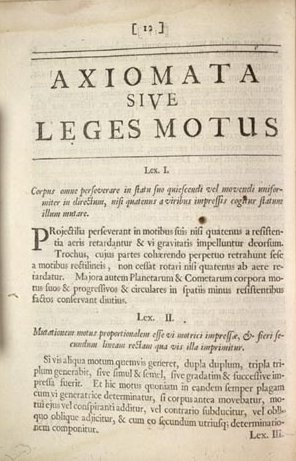Original publication of the first two laws, in Latin

Isaac Newton (1642–1727), the father of the study of dynamics – the study of motion – developed three sets of laws that are believed to be true because the results of tests done by scientists agree with the laws he produced.

## First law

• If a body is at rest it remains at rest or, if it is in motion, it moves with uniform velocity, until it is acted on by a resultant force.

A "uniform velocity" means that an object moves at a constant speed without changing direction (i.e. in a straight line). A "resultant force" means that the forces acting on the object are not balanced. In other words, the first law states two things:

1. A stationary object will only move if there is an unbalanced force acting on it.
2. A moving object will only change speed or direction if there is an unbalanced force acting on it.

The first part states that a table resting on the ground will not move unless pushed. Although gravity is acting on the table, pulling it down, there is a reaction force from the ground which is pushing it back. The forces acting on the table are balanced, so the object will not move.

The second part is harder to understand. A ball rolling along a flat surface will slow down and eventually come to a halt. But this is because of friction, which is a force that slows the ball down. A ball rolling down a slope is also affected by friction, but the force of gravity that causes it to move is stronger. In a place without the forces of friction, air resistance and gravity (e.g. in outer space) a moving object would keep on moving in a straight line if there were no force to slow it down or change its direction.

## Second law

This law provides the definition and calculation of force through mass and acceleration. Newton's second law says that acceleration is dependent on the forces acting upon an object and the mass of the object. Therefore if the force is increased, the acceleration is increased. And the more mass the object has, the acceleration decreases.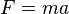$F = ma$

For example, weight is a force that we feel on Earth, caused by the gravity. Weight is calculated as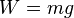$W = mg$

where m is the mass of the object and g is the local gravitational acceleration (not to be confused with G, the universal gravitational constant), roughly equal to 9.8 meters per second2 (32 feet per second2) on Earth.

We can express Newton's second law in terms of momentum. The momentum p of a particle is defined as the product of its mass m and velocity v: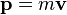$\mathbf{p} = m \mathbf{v}$

Newton's second law states that

• the resultant force acting on a particle equals the time rate of change of momentum of the particle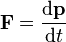$\mathbf{F} = \frac{\mathrm{d}\mathbf{p}}{\mathrm{d}t}$

For a particle of fixed mass (constant m),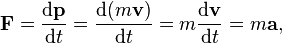$\mathbf{F} = \frac{\mathrm{d}\mathbf{p}}{\mathrm{d}t} = \frac{\mathrm{d}(m\mathbf v)}{\mathrm{d}t} = m\frac{\mathrm{d}\mathbf v}{\mathrm{d}t} = m\mathbf{a},$

which is the F = ma equation above in vector form.

## Third law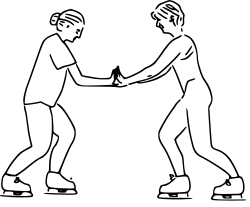Newton's third law. The skaters' forces on each other are equal in magnitude, and in opposite directions
• For every action, there is an equal and opposite reaction. Or every action always reacts in the opposite direction.

This is best understood with billiard balls, where you can easily see the action/reaction pairs of forces. Likewise, when you kick a football, not only does the ball move, but you feel a force on your foot.

The size of the forces on the first object equals the size of the force on the second object. The direction of the force on the first object is opposite to the direction of the force on the second object. Forces always come in pairs - equal and opposite action-reaction force pairs.

A variety of action-reaction force pairs are evident in nature. Consider the propulsion of a fish through the water. A fish uses its fins to push water backwards. But a push on the water will only serve to accelerate the water. Since forces result from mutual interactions, the water must also be pushing the fish forwards, propelling the fish through the water. The size of the force on the water equals the size of the force on the fish; the direction of the force on the water (backwards) is opposite the direction of the force on the fish (forwards). For every action, there is an equal (in size) and opposite (in direction) reaction force. Action-reaction force pairs make it possible for fish to swim.

Consider the motion of a car on the way to school. A car has wheels which spin forwards. As the wheels spin forwards, they grip the road and push the road backwards. Since forces result from mutual interactions, the road must also be pushing the wheels forward. The size of the force on the road equals the size of the force on the wheels (or car); the direction of the force on the road (backwards) is opposite the direction of the force on the wheels (forwards). For every action, there is an equal (in size) and opposite (in direction) reaction. Action-reaction force pairs make it possible for cars to move along a roadway surface.

## Related pagesNewton's laws of motion Facts for Kids. Kiddle Encyclopedia.